Foxtable(狐表)用户栏目专家坐堂 → 成绩排名

共有661人关注过本帖树形打印复制链接

# 主题：成绩排名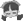21楼 | 信息 | 搜索 | 邮箱 | 主页 | UC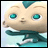加好友发短信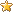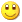Post By：2020/1/9 8:45:00 [只看该作者]

 谢谢老师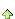22楼 | 信息 | 搜索 | 邮箱 | 主页 | UC加好友发短信Post By：2020/1/9 11:35:00 [只看该作者]

 Dim t As Table = e.Form.controls("Table1").Tablee.Form.controls("NavBar1").SelectedPage.ButtDim SQL As String ="Sele ct  c.学期,班级,考试名称,c.学生编号,学生姓名,学科,学生分数 Fr om ({学生基本信息} a Inner JOIN {学生缴费} b ON b.学生编号 = a.学生编号) Inner JOIN {学生成绩} c ON c.学期 = b.学期 And c.学生编号 = b.学生编号 Where c.学期 = '20191'"Dim cmd As New SQLCommandcmd.CommandText = SQLDim dt As DataTabledt = cmd.ExecuteReader() Dim b As New CrossTableBuilder("统计表1", dt)b.HGroups.AddDef("学期")b.HGroups.AddDef("考试名称")b.HGroups.AddDef("班级")b.HGroups.AddDef("学生编号")b.HGroups.AddDef("学生姓名")b.VGroups.AddDef("学科","{0}_分数")b.Totals.AddDef("学生分数", "学生分数")t.DataSource = b.BuildDataSource()Dim lst As new List(of String)dt = DataTables("成绩分析_Table1")For Each dc As DataCol In dt.DataCols    Dim Tel As String = dc.Caption    If Tel.Contains("_分数") Then        Dim Parts() As String = Tel.Split("_")        lst.add(Parts(0) & "_名次")    End IfNextDim idx As Integer= 6For Each s As String In lst    dt.DataCols.Add(s, Gettype(Double))    t.cols(s).move(idx)    idx += 2Next 还要 求老师，我想按班级、学科排名次，求代码，谢谢 [此贴子已经被作者于2020/1/9 11:37:41编辑过]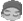23楼 | 信息 | 搜索 | 邮箱 | 主页 | UC加好友发短信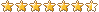Post By：2020/1/9 11:57:00 [只看该作者]24楼 | 信息 | 搜索 | 邮箱 | 主页 | UC加好友发短信Post By：2020/1/9 12:03:00 [只看该作者]

 Dim drs As List(Of DataRow) = DataTables("成绩表").Select("[班级] = " & bj, "总分 DESC")    For n As integer = 0 To drs.Count - 1 '遍历所有行        If n > 0 AndAlso drs(n)("总分") = drs(n-1)("总分") Then '如果总分和上一行相同            drs(n)("总分排名") = drs(n-1)("总分排名") '则排名等于上一行        Else            drs(n)("总分排名") = n + 1 '设置排名        End If    NextNext   老师，这段代码只是总分一列排名，我要多列排名。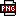此主题相关图片如下：qq截图20200109120921.png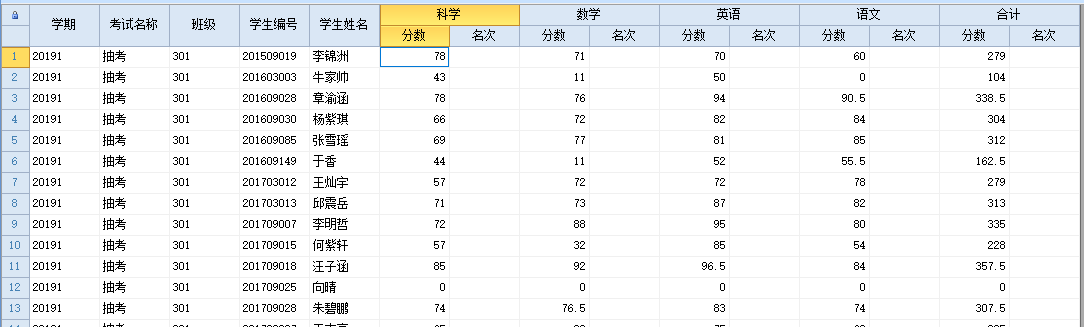[此贴子已经被作者于2020/1/9 12:08:33编辑过]25楼 | 信息 | 搜索 | 邮箱 | 主页 | UC加好友发短信Post By：2020/1/9 13:41:00 [只看该作者]

 每个列都调用同样的代码就行了Dim dict As new Dictionary(of String,String)For Each dc As DataCol In dt.DataCols    If dc.Caption.Contains("_分数") Then        dict.add(dc.Caption,dc.Name)    End IfNextfor each key as string in dict.keysdim cn as string = dict(key)dim pm as string = key.replace("_分数","_名次")Dim drs As List(Of DataRow) = dt.Select("[班级] = " & bj, cn & " DESC")    For n As integer = 0 To drs.Count - 1 '遍历所有行        If n > 0 AndAlso drs(n)(cn) = drs(n-1)(cn) Then '如果总分和上一行相同            drs(n)(pm) = drs(n-1)(pm) '则排名等于上一行        Else            drs(n)(pm) = n + 1 '设置排名        End If    NextNext26楼 | 信息 | 搜索 | 邮箱 | 主页 | UC加好友发短信Post By：2020/1/9 14:39:00 [只看该作者]

 下载信息  [文件大小：   下载次数： ]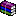点击浏览该文件:学生成绩排名.rar

[此贴子已经被作者于2020/1/9 14:59:22编辑过]27楼 | 信息 | 搜索 | 邮箱 | 主页 | UC加好友发短信Post By：2020/1/9 15:43:00 [只看该作者]

 Dim dict As new Dictionary(of String,String)For Each dc As DataCol In dt.DataCols    If dc.Caption> "" AndAlso dc.Caption.Contains("_分数") Then        dict.add(dc.Caption,dc.Name)    End IfNextFor Each bj As String In dt.GetValues("班级")    For Each key As String In dict.keys        Dim cn As String = dict(key)        Dim pm As String = key.replace("_分数","_名次")        Dim drs As List(Of DataRow) = dt.Select("[班级] = '" & bj & "'",cn & " DESC")        For n As Integer = 0 To drs.Count - 1 '遍历所有行            If n > 0 AndAlso drs(n)(cn) = drs(n-1)(cn) Then '如果总分和上一行相同                drs(n)(pm) = drs(n-1)(pm) '则排名等于上一行            Else                drs(n)(pm) = n + 1 '设置排名            End If        Next    NextNext28楼 | 信息 | 搜索 | 邮箱 | 主页 | UC加好友发短信Post By：2020/1/10 8:45:00 [只看该作者]

 谢谢总数 28 上一页 1 2 3